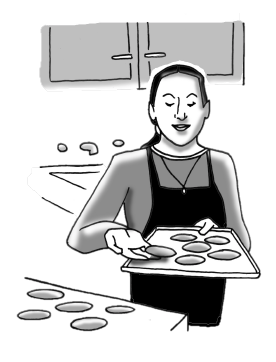### Home > AC > Chapter 4 > Lesson 4.1.6 > Problem4-58

4-58.Ms. B is making snickerdoodle cookies. Her recipe uses one-and-a-half teaspoons of cinnamon to make two-dozen cookies. If she needs to make thirteen-dozen cookies to give one cookie to each of her students, how much cinnamon will she need?

Use proportions to solve this problem. Assign a variable to the unknown number of teaspoons of cinnamon.
For this case, you can use the variable $n$

$\frac{ 1 \frac{ 1 }{2 } \text{ teaspoons}}{ 2\text{ dozen cookies}}= \frac{ n\text{ teaspoons}}{ 13\text{ dozen cookies}}$

Multiply both sides by $2$.

$\frac{ 1 \frac{ 1 }{2 } }{2 }= \frac{ n }{13 }$

Multiply both sides by $13$.

$1 \frac{ 1 }{2 } = \frac{ 2n }{13 }$

Divide by $2$ on both sides.

$19.5=2n$

Remember to use the conversion factor to determine how many tablespoons and teaspoons Ms. B will need.

$n=9.75 \text{ or }9 \frac{3}{4} \text{ teaspoons}$